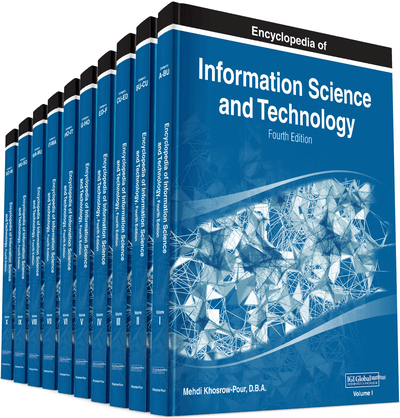# N-Tuple Algebra as a Unifying System to Process Data and Knowledge

Boris Alexandrovich Kulik (Institute of Problems in Mechanical Engineering RAS, Russia) and Alexander Yakovlevich Fridman (Institute for Informatics and Mathematical Modelling, Kola Science Centre of RAS, Russia)
DOI: 10.4018/978-1-5225-2255-3.ch173

## Abstract

Information technologies for analysis and processing heterogeneous data often face the necessity to unify representation of such data. To solve this problem, it seems reasonable to search for a universal structure that would allow for reducing different formats of data and knowledge to a single mathematical model with unitized manipulation methods. The concept of relation looks very prospective in this sense. So, with a view to developing a general theory of relations, the authors propose n-tuple algebra (NTA) developed as a theoretical generalization of structures and methods applicable in intelligence systems. NTA allows for formalizing a wide set of logical problems (deductive, abductive and modified reasoning, modeling uncertainties and so on).
Chapter Preview
Top

## N-Tuple Algebra: Basics And Features

N-tuple algebra is a mathematical system to deal with arbitrary n-ary relations. In NTA, such relations can be expressed as four types of structures called NTA objects. Every NTA object is immersed into a certain space of attributes. Names of NTA objects contain an identifier followed by a sequence of attributes names in square brackets; these attributes determine the relation diagram in which the NTA object is defined. For example, R[XYZ] denotes an NTA object defined within the space of attributes

## Key Terms in this Chapter

D-System: A set of homotypic D - n -tuples equal to the intersection of these D - n -tuples.

C-n-Tuple: An n -tuple of components defined in a certain relation diagram; domain of each component is a subset of the domain of the corresponding attribute.

Collisions: Situations occurring during defeasible reasoning when some new knowledge (hypothesis) is inputted. Such situations can be recognized as violations of some formally expressed rules and/or limitations which control consistency and meaning content of a logical system.

D-n-Tuple: An n -tuple of components enclosed in reversed square brackets and equal to a diagonal C -system with the same diagonal components.

N-Tuple Algebra: An algebraic system whose support is an arbitrary set of n -ary relations expressed by specific structures, namely, C - n -tuple, C -system, D - n -tuple, and D -system. These structures provide a compact expression for sets of elementary n -tuples.

C-System: A set of homotypic C - n -tuples that are denoted as a matrix in square brackets and equal to the intersection of these C - n -tuples. The rows of this matrix are C - n -tuples.

Generalized Operations and Relations: They differ from similar operations and relations of algebra of sets by the only feature: NTA objects (operands) are reduced to the same relation diagram before executing these operations or checking the relations.

## Complete Chapter List

Search this Book:
Reset